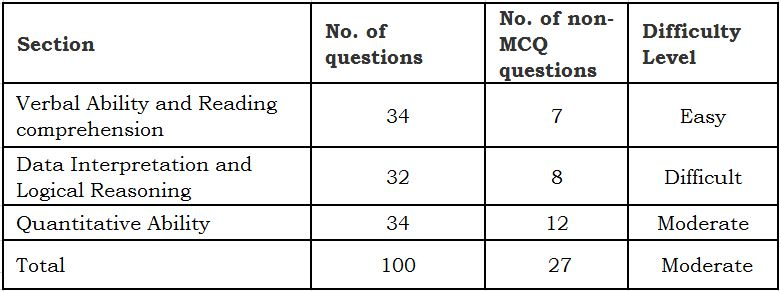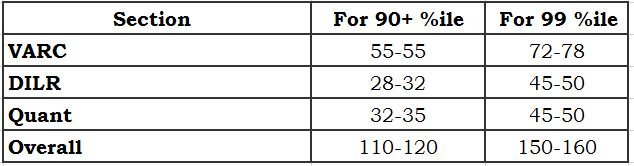Select PageAs it was widely anticipated, CAT-2018 did throw up any big surprise in term of pattern of the exam and nature of questions across the two slots. A slight change was observed in the nature of QA (Quantitative Ability) questions as a good number of questions in this section were lengthy and one extra non-MCQ questions i.e. 12 against to 11 in CAT 2017. The patter of CAT-2018 is shown below.In term of the total number of questions, the number of questions section-wise, total time (3 hours), sectional time (one hour for each section), difficulty level-wise, by and large, CAT 2018 was very similar to CAT 2017.
Marking scheme remained the same as it was in CAT-2017: each correct answer carried 3 marks, whereas each wrong answer fetched a penalty of -1 marks; there was no penalty for non-MCQ questions.
Since the patter and difficulty level, by and large, of the two slots of CAT-2018 was similar, we will take a holistic approach while analyzing the exam at sectional level. Section-wise analysis of CAT-2018 is given below.

Verbal Ability and Reading comprehension:

• The section was pretty much similar to that of CAT-2017 with only difference of 4 passages had 5 questions each and one had 4 questions in comparison to 3 passage with 6 questions each and 2 passage with 3 questions each in CAT 2017
• The difficulty level of the section remained, by and large, the same as it was in CAT-2017
• Reading comprehension passages were from an array of areas namely Economics, Sociology, History, Biology, Environment etc., and most of the questions, roughly 80-85%, could have been easily answered
• Question on Parajumbles, Summary and Odd-sentence were easy-to-moderate in nature

Data Interpretation and Logical Reasoning:

• By and large, this section was similar to that of the last year with 4 sets on DI and 4 on LR
• Difficulty level of the section was a little on lower side compared to that of the last couple of years
• DI questions weren’t calculation intensive
• LR sets were on familiar themes

Quantitative Ability

• The section was slightly difficult compared to that in CAT-2017, and this could have reduced the number of attempt in this section by 5-7 questions
• A significant number of questions were lengthy in nature making this section calculation and logic intensive.
• The number of Non-MCQ questions in the section went up by one compared to that in CAT-2017
• As it was the case in the last few years papers, the section was dominated by questions from Arithmetic followed by Algebra, Geometry and Modern Math. The number of questions from these areas was 12-14, 7-9, 6-8 and 3-4 respectively.

The following table shows a rough estimation of row scores required to achieve 90+ and 99+ %ile in CAT 2018, along with that in its individual sections: